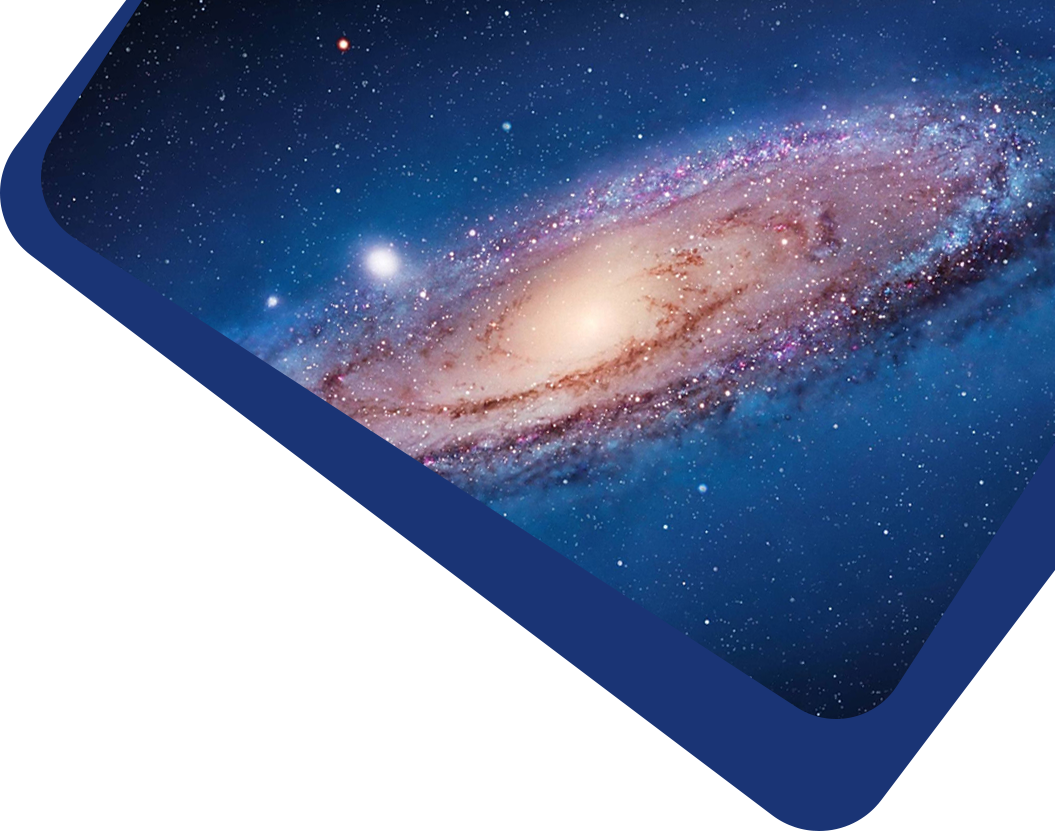Vol 22, No 11

## New Apsidal Motion in Four Eccentric Eclipsing Binaries: V610 Car, V944 Cep, V2815 Ori and V1260 Tau

#### Ahmet Bulut

Abstract

This paper presents an apsidal motion analysis. The apsidal motion analysis of the eccentric eclipsing binaries V610 Car (P = 4.84 days), V944 Cep (P = 6.56 days), V2815 Ori (P = 2.13 days), and V1260 Tau (P = 5.43 days) was performed using minima times from the O−C Gateway database, ASAS-3, ASAS-SN, and TESS, following Lacyʼs approach. The rates of change of periastron longitude were found to be 0.0253 ± 0.0041, 0.0347 ± 0.0015, 0.0288 ± 0.0010 and 0.0294 ± 0°.0079 cycle−1, respectively. From the light curve study, the first photometric mass ratio of V944 Cep was found to be q = 1.4994 ± 0.0012. A new light curve of V944 Cep was obtained using TESS data. The first absolute dimensions of V944 Cep were M1 = 1.10 ± 0.01 $$M_{\odot}$$, M2 = 1.64 ± 0.02 $$M_{\odot}$$, R1 = 2.04 ± 0.37 $$R_{\odot}$$, R2 = 3.77 ± 0.67 $$R_{\odot}$$ for the hotter primary component (T1 = 4925 K), and M1 = 1.04 ± 0.01 $$M_{\odot}$$, M2 = 1.57 ± 0.02 $$M_{\odot}$$, R1 = 2.26 ± 0.36 $$R_{\odot}$$, R2 = 3.74 ± 0.59 $$R_{\odot}$$ for the cooler primary component (T1 = 4475 K). For V610 Car, V944 Cep, V2815 Ori and V1260 Tau, the apsidal motion periods were found to be 188 ± 26 yr, 186 ± 8 yr, 73 ± 3 yr and 182 ± 38 yr, respectively. For V944 Cep, the internal structural constants of the component stars logk2,obs = −2.05 ± 0.01 and logk2,theo = −1.83 for hotter primary component and logk2,obs = −1.97 ± 0.01 and logk2,theo = −1.89 for cooler primary component were calculated.

Keywords

(stars:) binaries: eclipsing – stars: fundamental parameters – techniques: photometric

Full Text
Refbacks